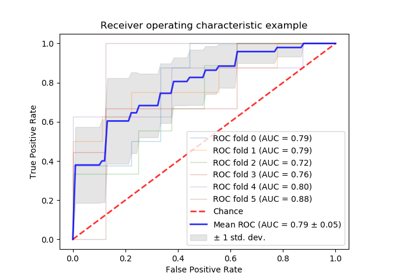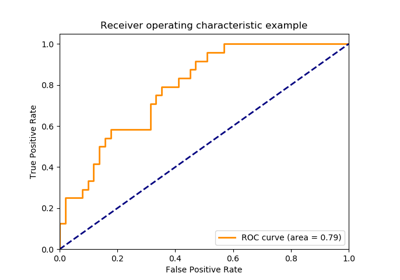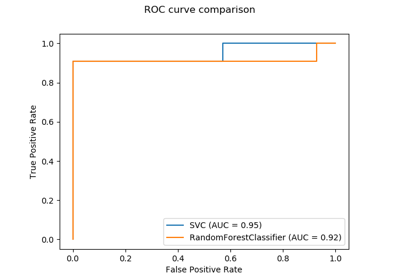sklearn.metrics.roc_auc_score¶

sklearn.metrics.roc_auc_score(y_true, y_score, average='macro', sample_weight=None, max_fpr=None, multi_class='raise', labels=None)[source]

Compute Area Under the Receiver Operating Characteristic Curve (ROC AUC) from prediction scores.

Note: this implementation can be used with binary, multiclass and multilabel classification, but some restrictions apply (see Parameters).

Read more in the User Guide.

Parameters
y_truearray-like of shape (n_samples,) or (n_samples, n_classes)

True labels or binary label indicators. The binary and multiclass cases expect labels with shape (n_samples,) while the multilabel case expects binary label indicators with shape (n_samples, n_classes).

y_scorearray-like of shape (n_samples,) or (n_samples, n_classes)

Target scores. In the binary and multilabel cases, these can be either probability estimates or non-thresholded decision values (as returned by decision_function on some classifiers). In the multiclass case, these must be probability estimates which sum to 1. The binary case expects a shape (n_samples,), and the scores must be the scores of the class with the greater label. The multiclass and multilabel cases expect a shape (n_samples, n_classes). In the multiclass case, the order of the class scores must correspond to the order of labels, if provided, or else to the numerical or lexicographical order of the labels in y_true.

average{‘micro’, ‘macro’, ‘samples’, ‘weighted’} or None, default=’macro’

If None, the scores for each class are returned. Otherwise, this determines the type of averaging performed on the data: Note: multiclass ROC AUC currently only handles the ‘macro’ and ‘weighted’ averages.

'micro':

Calculate metrics globally by considering each element of the label indicator matrix as a label.

'macro':

Calculate metrics for each label, and find their unweighted mean. This does not take label imbalance into account.

'weighted':

Calculate metrics for each label, and find their average, weighted by support (the number of true instances for each label).

'samples':

Calculate metrics for each instance, and find their average.

Will be ignored when y_true is binary.

sample_weightarray-like of shape (n_samples,), default=None

Sample weights.

max_fprfloat > 0 and <= 1, default=None

If not None, the standardized partial AUC  over the range [0, max_fpr] is returned. For the multiclass case, max_fpr, should be either equal to None or 1.0 as AUC ROC partial computation currently is not supported for multiclass.

multi_class{‘raise’, ‘ovr’, ‘ovo’}, default=’raise’

Multiclass only. Determines the type of configuration to use. The default value raises an error, so either 'ovr' or 'ovo' must be passed explicitly.

'ovr':

Computes the AUC of each class against the rest  . This treats the multiclass case in the same way as the multilabel case. Sensitive to class imbalance even when average == 'macro', because class imbalance affects the composition of each of the ‘rest’ groupings.

'ovo':

Computes the average AUC of all possible pairwise combinations of classes . Insensitive to class imbalance when average == 'macro'.

labelsarray-like of shape (n_classes,), default=None

Multiclass only. List of labels that index the classes in y_score. If None, the numerical or lexicographical order of the labels in y_true is used.

Returns
aucfloat

average_precision_score

Area under the precision-recall curve

roc_curve

Compute Receiver operating characteristic (ROC) curve

References

1

Wikipedia entry for the Receiver operating characteristic

2

Analyzing a portion of the ROC curve. McClish, 1989

3

Provost, F., Domingos, P. (2000). Well-trained PETs: Improving probability estimation trees (Section 6.2), CeDER Working Paper #IS-00-04, Stern School of Business, New York University.

4

Fawcett, T. (2006). An introduction to ROC analysis. Pattern Recognition Letters, 27(8), 861-874.

5

Hand, D.J., Till, R.J. (2001). A Simple Generalisation of the Area Under the ROC Curve for Multiple Class Classification Problems. Machine Learning, 45(2), 171-186.

Examples

>>> import numpy as np
>>> from sklearn.metrics import roc_auc_score
>>> y_true = np.array([0, 0, 1, 1])
>>> y_scores = np.array([0.1, 0.4, 0.35, 0.8])
>>> roc_auc_score(y_true, y_scores)
0.75

Examples using sklearn.metrics.roc_auc_score¶